## Indefinite Integrals

The notation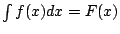means that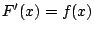on some (usually specified) domain of definition of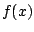.

Definition 2.2.1 (Anti-derivative)   We call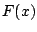an anti-derivative of.

Proposition 2.2.2   Suppose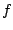is a continuous function on an interval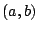. Then any two antiderivatives differ by a constant.

Proof. If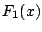and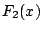are both antiderivatives of a function, then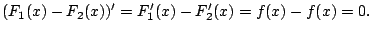Thus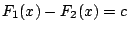from some constant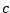(since only constant functions have slope 0 everywhere). Thus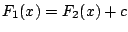as claimed.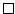We thus often write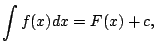whereis an (unspecified fixed) constant.

Note that the proposition need not be true ifis not defined on a whole interval. For example,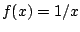is not defined at 0. For any pair of constants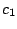,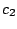, the function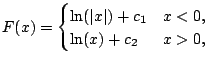satisfiesfor all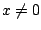. We often still just write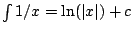anyways, meaning that this formula is supposed to hold only on one of the intervals on which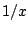is defined (e.g., on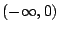or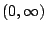).

We pause to emphasize the notation difference between definite and indefinite integration.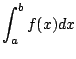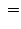a specific number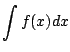a (family of) functions

One of the main goals of this course is to help you to get really good at computing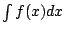for various functions. It is useful to memorize a table of examples (see, e.g., page 406 of Stewart), since often the trick to integration is to relate a given integral to a known one. Integration is like solving a puzzle or playing a game, and often you win by moving into a position where you know how to defeat your opponent, e.g., relating your integral to integrals that you already know how to do. If you know how to do a basic collection of integrals, it will be easier for you to see how to get to a known integral from an unknown one.

Whenever you successfully compute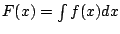, then you've constructed a mathematical gadget that allows you to very quickly compute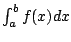for any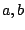(in the interval of definition of). The gadget is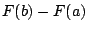. This is really powerful.

William Stein 2006-03-15